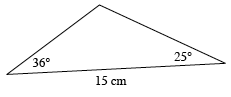### Home > PC > Chapter 3 > Lesson 3.2.2 > Problem3-77

3-77.

Find the area of the triangle shown below.Use the Law of Cosines to determine the length of one of the missing sides.

where $θ$ is the angle between sides $a$ and $b$